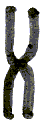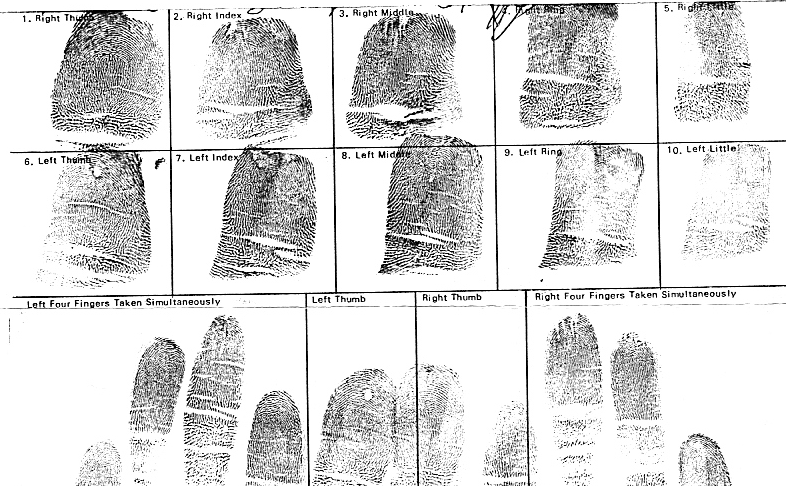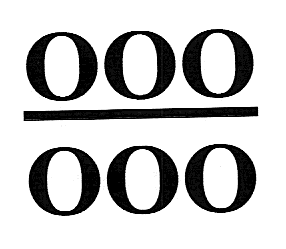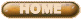ANDRES J. WASHINGTON'S RESEARCH

Even though this research may not be directly related to the Great Pyramid, it is related to modern Egyptology research.  It is known that well preserved mummies still exhibit fingerprint patterns.  Besides DNA analysis, fingerprints help us in determining some genetic features and family relationships of mummies.  It is another ongoing research tool in our understanding of ancient Egypt.  Andres Washington is one of the leading fingerprint experts in the world with over 20 years professional experience.  He has worked for agencies and has been a consultant for many important fingerprint studies.  We hope you enjoy this section on his research and interests.

Andres J. Washington
Fingerprint Geometric Analysis

Ridge Dissociation

Figure "H" displays a rare and severe degree of ridge dissociation in which all ten digits are affected. It can be noted that the entire set of fingerprints display an extreme narrowing of ridges in the center of the pattern area. The ridge events mostly encountered in various but somewhat uniform sequencing are ridge dots, enclosures and bifurcations. Many of the bifurcations recurve in an unconventional manner with appendages between the shoulders that extend to form other recurves. In some cases there is also the rare event of crossing ridges that resemble the configuration of chromosomes.Figure "H" displays this event in a vertical formation.

Researchers have discovered ridge dissociation to occur in some individuals suffering from schizophrenia as well as physical conditions such as epilepsy, mongolism and albinism.

Figure "H"NCIC FPC Filing Sequence Formula

Each code for the NCIC FPC is assigned a number of the 115 possible:

 PI = 0.1 17 = 2.9 45 = 5.7 73 = 8.5 AA = 11.3 PM = 0.2 18 = 3 46 = 5.8 74 = 8.6 SR = 11.4 PO = 0.3 19 = 3.1 47 = 5.9 75 = 8.7 XX = 11.5 CI = 0.4 20 = 3.2 48 = 6 76 = 8.8 CM = 0.5 21 = 3.3 49 = 6.1 77 = 8.9 CO = 0.6 22 = 3.4 50 = 6.2 78 = 9 DI = 0.7 23 = 3.5 51 = 6.3 79 = 9.1 DM = 0.8 24 = 3.6 52 = 6.4 80 = 9.2 DO = 0.9 25 = 3.7 53 = 6.5 81 = 9.3 XI = 1 26 = 3.8 54 = 6.6 82 = 9.4 XM = 1.1 27 = 3.9 55 = 6.7 83 = 9.5 XO = 1.2 28 = 4 56 = 6.8 84 = 9.6 1 = 1.3 29 = 4.1 57 = 6.9 85 = 9.7 2 = 1.4 30 = 4.2 58 = 7 86 = 9.8 3 = 1.5 31 = 4.3 59 = 7.1 87 = 9.9 4 = 1.6 32 = 4.4 60 = 7.2 88 = 10 5 = 1.7 33 = 4.5 61 = 7.3 89 = 10.1 6 = 1.8 34 = 4.6 62 = 7.4 90 = 10.2 7 = 1.9 35 = 4.7 63 = 7.5 91 = 10.3 8 = 2 36 = 4.8 64 = 7.6 92 = 10.4 9 = 2.1 37 = 4.9 65 = 7.7 93 = 10.5 10 = 2.2 38 = 5 66 = 7.8 94 = 10.6 11 = 2.3 39 = 5.1 67 = 7.9 95 = 10.7 12 = 2.4 40 = 5.2 68 = 8 96 = 10.8 13 = 2.5 41 = 5.3 69 = 8.1 97 = 10.9 14 = 2.6 42 = 5.4 70 = 8.2 98 = 11 15 = 2.7 43 = 5.5 71 = 8.3 99 = 11.1 16 = 2.8 44 = 5.6 72 = 8.4 TT = 11.2

The NCIC FPC contains 20 characters, each pair of characters represents one digit beginning with the right thumb as the No. 1 digit and ending with the left little finger as the No. 10 digit; the left thumb is then No. 6. For every consecutive pair of characters, one code can be assigned out of a possible 115 codes (from PI to XX ). This means that there are actually only 10 individual segments for the NCIC FPC code , with each one maintaining a pair of the 20 characters to represent an individual code.

Let (X) equal the number of assigned to the NCIC FPC code.

Each of the ten segments with the exception of segment #1, must be assigned a decimal number which shall be added to the assigned number for the given NCIC FPC Code.

Digit 1 2 3 4 5 6 7 8 9 10

Number

Assigned X X.00001 X.00002 X.00003 X.00004 X.00005 X.00006 X.00007 X.00008 X.00009

This adding of the decimal number to the assigned number for the NCIC FPC code must be done in order to establish a unique numerical value to each segment of the ten-segment unit. Segment number 1, however, need not be assigned a decimal value because the other nine segments maintain an identity distinguishable from it. ( The reason for choosing #1 segment as the one which shall not be assigned a decimal value is because #1 segment is the only segment which can provide us with the lowest possible number in the calculation if no decimal was to be added to it. ) It can be noted that in this way no two or more segments can provide the same exact number. Furthermore, no two NCIC FPC codes   provide the same filing number, even if the original code appeared in a reverse sequence.

NCIC 14 XX AA CO 04 SR 59 TT DM 10

FPC

Code

Number 2.6 11.5 11.3 0.6 1.6 11.4 7.1 11.2 0.8 2.2

Assigned

To The Given

Code

Decimal 2.6 11.50001 11.30002 0.60003 1.60004 11.40005 7.10006 11.20007 0.80008 2.20009

Assigned

Number

After the decimal number is added to the assigned number for the NCIC FPC code, multiplication takes place. That is, #1 segment times #2 segment and that product multiplied by #3 segment, and so on until #10 segment has been included in the multiplication. The end product shall provide the filing location for the given NCIC FPC code.

When there are ten segments and 115 possible codes for each segment, the total number of possible combinations is approximately 4.04557E+20 or exactly 404,555,773,570,791,015,625.

Example: ( 2.6) X ( 11.50001 ) X ( 11.30002 ) X ( 0.60003 ) X ( 1.60004 ) X ( 11.40005 ) X ( 7.10006 ) X ( 11.20007 ) X ( 0.80008 ) X ( 2.20009 )

= 517,628.2327.

In conclusion, the NCIC FPC code 14XXAACO04SR59TTDM10

would be filed as the 517,628.2327th code between 1.00451E-10 and 40,457,160,428.

(Microsoft Excel 97 or later is required)

FINGERPRINT GEOMETRIC ANALYSIS
Andres J. Washington

Post Office Box 165

Bronx, NY 10451-0165

USA
Fax: 253 295-3335
E mail fingerprints@fcc.netFINGERPRINT DIAGONAL REVERSE SEQUENCE ARRANGEMENT

The original sequence found in section "A" is for the sixty-four possibilities when the # 2,3,4,7,8 and 9 fingers are considered; this sequence only includes the inner replica uhren online and outer loops. When a number is assigned to each box from  left to right and working down to
the last box # 64, box 64 would equal.

Due to the fact that the inked fingerprint is in actual reverse, (Mirroring) it has been determined that if the  sequence in section "A" was to be arranged to the sequence found in section "B" it would then cheap replica sunglasses be in reverse  displaying a different perspective of the total possibilities in relation to each other.  This diagonal reverse is then used to provide the arrangement of the Second Reference Sequence found in section "C".

The second reference sequence works as a verification in its outcome of how the first reference sequence was established.  If the first reference sequence was not in proper arrangement then the second reference sequence would not be in a uniform sequencing pattern.  A close examination of the fingerprint codes found in the second reference sequence reveals a definite pattern along a diagonal basis.

For further analysis, the second reference sequence has been divided into three areas;aaaaa replica watches named area A, area B, and area C.  In area "A" the extreme diagonal of each box contains its opposite code.  In area "B" and "C" the extreme diagonal of each box contains the same code in reverse.

(Microsoft Word 97 or later is required)

Andres J. Washington

www.dermatoglyphics.comAndres J. Washington
Fingerprint Geometric Analysis

The Fingerprints of Malcolm X

The right thumb impression of Malcolm X (1925-1965) is a radial loop, (the ridges enter and exit in the direction of the radius bone) which is horizontal.  I have never encountered a horizontal radial loop on the right thumb before.  Fingerprint studies on the frequency of patterns report radial loops to exist on the right thumb at frequencies not higher than one percent.  The left index fingerprint is also a horizontal radial loop.

The right ring finger of Malcolm is a central pocket loop whorl pattern. The frequency for this pattern to appear on the right ring finger is less than .250 percent.  This pattern is the only whorl pattern in the ten set of fingerprints displayed.

Under the original rules for classifying fingerprints according to the Henry System of Fingerprint Classification and Filing, we would conclude that there are three plain arch patterns, the right index, right middle and the left replica orologi italia thumb.  However, under revised rules for classification, the right middle finger is classified as a tented arch pattern (technical).  A technical tented arch pattern is a pattern that has two of the three requirements necessary to be considered a loop pattern.  This pattern (#3) has a delta and a core but does not display a recurving ridge in front of the delta.  I have not seen a study to include the frequency of technical tented arches on the right middle finger.  In reference to the classic tented arch, it's frequency on the right middle finger has not been higher than 3 percent.

The breakdown on the combination of fingerprint patterns for Malcolm X would be the following:

Four Ulnar Loop patterns (# 5,8,9 and 10)
Two Plain Arch patterns (# 2 and 6)
One Tented Arch Pattern (# 3)
Two Radial Loop patterns (# 1 and 7)
One Central Pocket Loop Type Whorl pattern (# 4)

An extraordinary configuration of the dermal ridge arrangements and the variance of pattern display indicates an elevated mental manifestation.